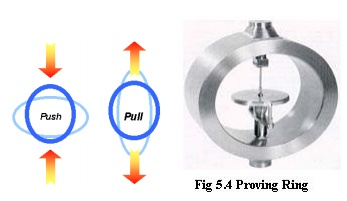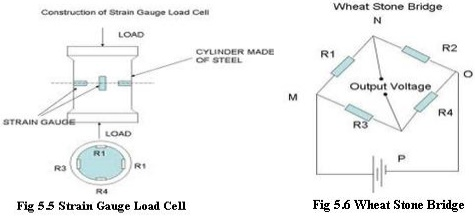Home | | Metrology and Measurements | Measurement of Force

# Measurement of Force

Force is a basic engineering parameter, the measurement of which can be done in many ways as follows: · Direct methods · Indirect methods

MEASUREMENT OF FORCE

The mechanical quantity which changes or tends to change the motion or shape of a body to which it is applied is called force. Force is a basic engineering parameter, the measurement of which can be done in many ways as follows:

·        Direct methods

·        Indirect methods

·        Direct methods

It involves a direct comparison with a known gravitational force on a standard mass, say by a balance.

· Indirect methods

It involves the measurement of effect of force on a body, such as acceleration of a body of known mass subjected to force.

Devices to measure Force

·        Scale and balances

a.     Equal arm balance

b.    Unequal arm balance

c.     Pendulum scale

·        Elastic force meter (Proving ring)

Scale and balances

a.  Equal arm balance

An equal arm balance works on the principle of moment comparison. The beam of the equal arm balance is in equilibrium position when,

Clockwise rotating moment = Anti-clockwise rotating moment M2L2 = M1L1

That is, the unknown force is balanced against the known gravitational force.

Description

The main parts of the arrangement are a follows:

·        A beam whose centre is pivoted and rests on the fulcrum of a knife edge. Either side of the beam is equal in length with respect to the fulcrum

·        A pointer is attached to the center of the beam. This pointer will point vertically downwards when the beam is in equilibrium.

·        A Provision to place masses at either end of the beam.Operation

•        A known standard mass (m1) is placed at one end of the beam and an unknown mass (m2) is placed at its other end.

• Equilibrium condition exists when,          clockwise rotating moment = Anti- clockwise rotating moment

·        Moreover at a given location, the ear masses (m1 and m2) and hence at equilibrium condition. W1=W2. That is, the

unknown force (weight) will be equal to the known force (weight).

b.    Unequal arm balance

An unequal arm balance works on the principle of moment comparison.

The beam of the unequal arm balance is in equilibrium position when,

Clockwise rotating moment = Anti-clockwise rotating moment

F x L2 = Fx x L1Description

The main parts of the arrangements are as follows:

·        A   graduated   beam   pivoted   to   a   knife   ed

·        A leveling pointer is attached to the beam

·        A known mass “m” is attached to the r unknown forcem”“F”can. Thisslidemasson the“ right

·        Provisions are made tox” applyontheanleftunknos beam.

Operation

·        An unknown force “Fx” is applied on t edge “Z” as shown

·        Now the positi”on theof massright“mside of th the leveling pointer reads null balance position. When the leveling pointer is

in null balance position, the beam is in equilibrium.

Clock wise rotating moment = Anti-clock wise rotating moment

Fx.L1 = F. L2

Fx = Mg.L2/L1

·        Thus the unknownx”is proportionalforce“F2”oftothethemad “m” from the knife edge “Y”

·        The right hand side of the beam which is graduated is calibrated to get a direct

measurex”  of     “F

c.  Pendulum Scale(Multi-lever Type)

It is a moment comparison device. The unknown force is converted to torque which is then balanced by the torque of a fixed standard mass arranged as a pendulum.Description

• The   scale’s   frames   carry   support   ribb These support ribbons are attached to the sectors. The loading ribbons are attached to the sectors and the load rod a shown. The load rod is inturn attached to the weighing platform.

• The two sectors are connected on either side of an equalizer beam. The sectors carry counter weighs. To the center of  the equalizer beam is attached a rack and pinion arrangement.

• A  pointer  is  attached  to  the  pinion  which  sweeps  over  a  weight  (force)  calibrated scale.

Operation

·        As the sectors rotate about the pivots, it moves the counter weights outwards, This movements increases the counter weight effective moment until the torque produced by the force applied to the load rod and the moment produced by the counter weight balance each other, thereby establishing an equilibrium.

·        During the process of establishing equilibrium, the equalizer beam would be displaced downwards. As the rack is attached to the equalizer beam, the rack also is displaced downwards rotating the pinion.

·        As the pointer is attached to the pinion, the rotation of the pinion makes the pointer to assume a new position on the scale. The scale is calibrated to read the weight directly. Thus the force applied on the load rod is measured.

Elastic force meter (Proving Ring)

When a steel ring is subjected to a force across its diameter, it deflects. This deflection is proportional to the applied force when calibrated.Description

A steel ring attached with external bosses to apply force. A precision micrometer with one of its ends mounted on a vibrating reed.

Operation

·        The force to be measured is applied to the external bosses of the proving ring. Due to the applied force, the ring changes in diameter. This deflection of the ring is proportional to the applied force.

·        At this stage, the reed is plucked to obtain a vibrating motion. When the reed is vibrating, the micrometer wheel is turned until the micrometer contact moves forward and makes a noticeable damping of the vibrating reed.

·        Now the micrometer reading is noted which is a measure of deflection of the ring. The device is calibrated to get a measure of force in terms of deflection of the proving ring.·        When a steel cylinder is subjected to a force, it tends to change in dimension. On this cylinder if strain gauges are bonded, the strain gauge also is stretched or compressed, causing a change in its length and diameter.

·        This change in dimension of the strain gauge causes its resistance to change.

This  change  in  resistance  of  the  strain  gauge  becomes  a  measure  of  the

applied force.

Description

· A cylinder made of steel on which four identical strain gauges are mounted.

·        Out of the four strain gauges, two of them (R1 and R4) are mounted along the direction of the applied load(Vertical gauges)

·        The other tow strain gauges (R2 and R3 horizontal gauges) are mounted circumferentially at right angles to gauges R1 and R4.

·                   The four gauges are connected to the four limbs of wheat stone bridge. Operation

·        When there is no load on the steel cylinder, all the four gauges will have the same resistance. As the terminals N and P are at the same potential, the wheat stone bridge is balanced and hence the output voltage will be zero.

·        Now the force to be measured is applied on the steel cylinder. Due to this, the vertical gauges R1 and R4 will under go compression and hence there will be a decrease in resistance. At the same time, the horizontal gauges R2 and R3 will undergo tension and there will be an increase in resistance. Thus when strained, the resistance of the various gauges change.

·        Now the terminals N and P will be at different potential and the change in output voltage due to the applied load becomes a measure of the applied load when calibrated.

·        When a force is applied on liquid medium contained in a confined space, the pressure of the liquid increases. This increase in pressure of the liquid is proportional to the applied force. Hence a measure of the increase in pressure of the liquid becomes a measure of the applied force when calibrated.

•        The force to be measure is applied to the piston•        The applied force moves the piston down wards and deflects the diaphragm and this deflection of the diaphragm increase the pressure in the liquid medium.

•        This increase in pressure of the liquid medium is proportional to the applied force. This increase in pressure is measured by the pressure gauge which is connected to the liquid medium.

•        The pressure is calibrated in force units and hence the indication in the pressure gauge becomes a measure of the force applied on the piston.

•        If a force is applied to one side of a diaphragm and an air pressure is applied to the other side, some particular value of pressure will be necessary to exactly balance the force. This pressure is proportional to the applied force.

•        The force to be measured is applied to the top side of the diaphragm. Due to this  force, the diaphragm deflects and causes the flapper to shut-off the nozzle opening.

•        Air supply is provided at the bottom of the diaphragm. As the flapper closes the nozzle opening, a back pressure results underneath the diaphragm.·        This back pressure acts on the diaphragm producing an upward force. Air pressure is regulated until the diaphragm returns to the pre-loaded position which is indicated by air which comes out of the nozzle.

·        At this stage, the corresponding pressure indicated by the pressure gauge becomes a measure of the applied force when calibrated.

Study Material, Lecturing Notes, Assignment, Reference, Wiki description explanation, brief detail
Mechanical : Metrology and Measurements : Measurement of Power, Flow and Temperature Related Properties : Measurement of Force |